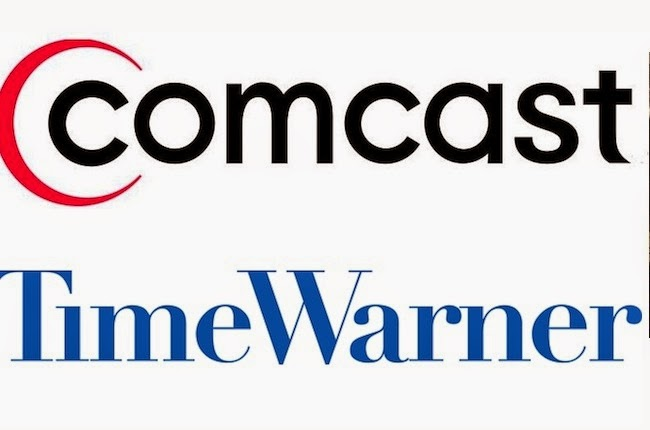## Thursday, April 10, 2014

### 74 | The Comcast CoincidenceNotice the "double C" in the Comcast logo.  CC = 33

The cable television conglomerate Comcast, who has recently purchased NBC and Time Warner and become the biggest multimedia provider in the Milky Way galaxy, has 74 numerology.
• Comcast = 3+15+13+3+1+19+20 = 74
• Time from "Time Warner" is inverse of Comcast
• Time = 20+9+13+5 = 47
Other words with 74 numerology are as follows:
• Tempt = 20+5+13+16+20 = 74
• Jesus = 10+5+19+21+19 = 74
• Cross = 3+18+15+19+19 = 74
• Lucifer = 12+21+3+9+6+5+18 = 74
Then there is also the 33rd state, Oregon, which ties in with Jesus being crucified at the age of 33.  It has an abbreviation of OR.
• OR = 15+18 = 33
• Oregon = 15+18+5+7+15+14 = 74
Comcast's headquarters are located in Philadelphia, Pennsylvania, the "Keystone" state, and at the address 1701 John F. Kennedy Blvd.  The following is a list of the key people running Comcast.

• Keystone = 2+5+7+1+2+6+5+5 = 33
• Brian = 2+18+9+1+14 = 44
• Michael = 4+9+3+8+1+5+3 = 33
• David = 4+1+4+9+4 = 22
• All names have master numerology (multiples of 11)
*While writing this post, a Comcast salesman came to my door, and I ended up telling him about this blog and this post I am writing.  We just finished talking after 3-hours, and I even signed up for Comcast to help him out.  I told him it was only because I didn't believe in coincidences, and I figure by next year, or maybe two years from now, Comcast will own everything anyway.  We both laughed.

Life is strange.  I think I have a new friend.  And he works for Comcast.